2019/01/28

# 基本概念

## 第一型及第二型错误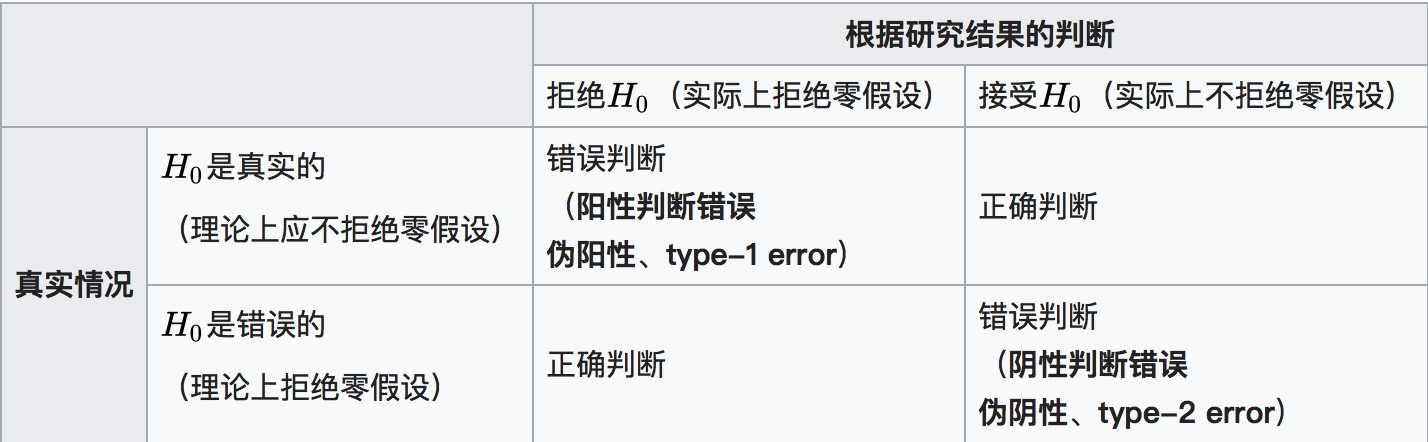# 基本原理

## 带概率性质的反证法原理

1. 假设结论的反面成立，即反设；
2. 由这个假设出发，经过正确的推理导出矛盾，即归谬；
3. 由矛盾判定假设不正确，从而肯定命题结论正确，即结论。

• 第一步，反设：如果$$\sqrt{2}$$是有理数，则必有：$$\sqrt { 2 } = \frac { p } { q }$$，其中p,q是互质的正整数。
• 第二步，归谬：两边平方，则有： \begin{aligned} 2 & = \frac { p ^ { 2 } } { q ^ { 2 } } \\ p ^ { 2 } & = 2 q ^ { 2 } \end{aligned} 则$$p$$为偶数，设$$p=2r$$，则有： $$\begin{array} { c } { 4 r ^ { 2 } = 2 q ^ { 2 } } \\ { 2 r ^ { 2 } = q ^ { 2 } } \end{array}$$ 则$$q$$也是偶数，这与p、q互质矛盾。
• 第三步，结论：因此假设不成立，$$\sqrt{2}$$是无理数。

## 小概率事件原理

0.05是足够小的概率3，一般认为，在一次抽样(试验)中，小概率事件几乎不可能发生，如果出现发生了，则说明事先的假设是错误的。但小概率事件并不是一定不会发生，当抽样次数足够多时，小概率事件是一定会发生的。这说明即使是一次抽样，小概率事件仍有可能发生，也就是说存在判断错误的可能性。

### 显著性水平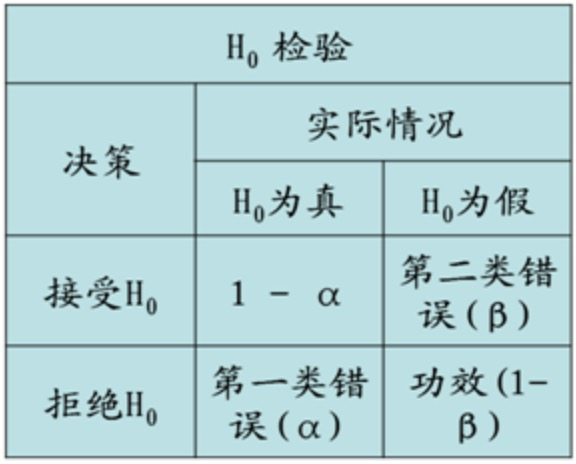# 假设检验步骤

1. 最初研究假设为真相不明。
2. 第一步是提出相关的零假设和备择假设。这是很重要的，因为错误陈述假设会导致后面的过程变得混乱。
3. 第二步是考虑检验中对样本做出的统计假设；例如，关于独立性的假设或关于观测数据的分布的形式的假设。这个步骤也同样重要，因为无效的假设将意味着试验的结果是无效的。
4. 决定哪个检测是合适的，并确定相关检验统计量 T。
5. 在零假设下推导检验统计量的分布。在标准情况下应该会得出一个熟知的结果。比如检验统计量可能会符合t-分布或正态分布。
6. 选择一个显著性水平 (α)，若低于这个概率阈值，就会拒绝零假设。最常用的是 5% 和 1%。
7. 根据在零假设成立时的检验统计量T分布，找到数值最接近备择假设，且机率为显著性水平 (α)的区域，此区域称为“拒绝域”，意思是在零假设成立的前提下，落在拒绝域的机率只有α。
8. 针对检验统计量T，根据样本计算其估计值tobs
9. 若估计值tobs未落在“拒绝域”，接受零假设。若估计值tobs落在“拒绝域”，拒绝零假设，接受备择假设。

# 比较分析

## 比较分析有哪些种？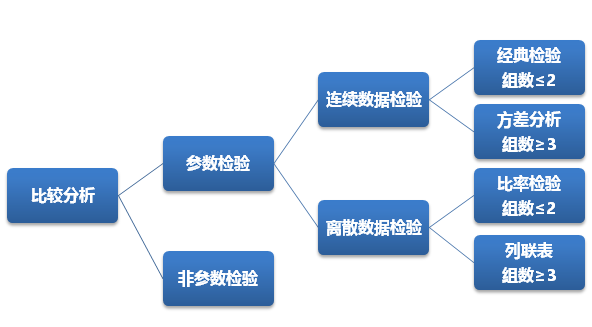• 经典比较：包括大家非常熟悉的$$Z$$-检验、$$t$$-检验、$$\chi ^{2}$$检验、$$F$$-检验。
• 方差分析：包含的内容实在是太广泛了，而针对比较分析来说，一般是指单因子和两因子方差分析。
• 比率检验：主要是单样本或两样本服从二项分布和泊松分布的比率检验。
• 列联表：常用的就是大家熟知的R*C表了，当然还有很复杂的应用。
• 非参数检验：统计学家们为不服从特定分布的数据研发了很多检验方法。

## T检验

• 单样本检验：检验一个正态分布的总体的均值是否在满足零假设的值之内（样本均数与已知总体均数的比较），例如检验一群军校男生的身高的平均是否符合全国标准的170公分界线。

适用条件：

• 样本来自于正态分布的群体（当样本量足够大，即使原数据不服从正态分布，由中心极限定理可知，其样本均数的抽样分布仍然是正态的。因此当样本量较大时，研究者很少去考虑单样本t检验的适用条件，此时真正会限制该方法使用的是均数是否能够代表相应数据的集中趋势，只要数据分布不是强烈的偏态，一般而言单样本t检验都是适用的。）
• 样本量较小（通常 n < 30，n较小时，一般要求样本取自正态总体）
• 总体标准差未知的情况

检验零假说为一群来自正态分配独立样本$$x_i$$之总体期望值$$\mu$$为$$\mu_0$$可利用以下统计量

$t = \frac { \overline { x } - \mu _ { 0 } } { s / \sqrt { n } }$

其中$$i = 1 \dots n$$，$$\overline { x } = \frac { \sum _ { i = 1 } ^ { n } x _ { i } } { n }$$为样本平均数，$$s = \sqrt { \frac { \sum _ { i = 1 } ^ { n } \left( x _ { i } - \overline { x } \right) ^ { 2 } } { n - 1 } }$$为样本标准偏差，$$n$$为样本数。该统计量$$t$$在零假说：$$\mu = \mu _ { 0 }$$为真的条件下服从自由度为$$n − 1$$的$$t$$分布。

大量检测已知正常人血浆载脂蛋白E（ apo E）总体平均水平为4.15mmol/L。某医师经抽样测得41例陈旧性心机梗死患者的血浆载脂蛋白E平均浓度为5.22mmol/L，标准差为1.61mmol/L。据此能否认为陈旧性心肌梗死患者的血浆载脂蛋白E平均浓度与正常人的平均浓度不一致？

1. 建立假设、确定检验水准α=0.05，双侧检验，自由度为 40-1 = 40 H0：μ = μ0 （零假设null hypothesis，即与正常人浓度一致） H1：μ ≠ μ0（备择假设alternative hypothesis，即与正常人浓度不一致）
2. 计算检验统计量，根据上面的公式计算得$$t = \frac { \overline { x } - \mu _ { 0 } } { s _ { \overline { x } } } = \frac { \overline { x } - \mu _ { 0 } } { s / \sqrt { n } } = \frac { 5.22 - 4.15 } { 1.61 / \sqrt { 41 } } = 4.26$$
3. 确定p值和作出推断结论。查t分布表7， t0.05/2,40=2.021， t=4.26>t0.05/2,40，p < 0.05（如下图，t分布在t>0侧为减函数）。按α=0.05水准，拒绝H0，接受H1，可认为陈旧性心肌梗死患者的血浆载脂蛋白E平均浓度与正常人的差别有统计学意义，结合专业可以认为前者平均浓度较高。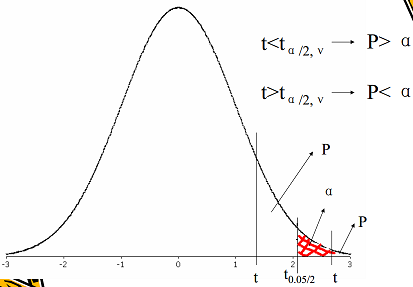• 双样本检验：其零假设为两个正态分布的总体的均值之差为某实数，例如检验二群人的身高之平均是否相等。这一检验通常被称为学生t检验。但更为严格地说，只有两个总体的方差是相等的情况下（方差齐性8，才称为学生t检验；否则，有时被称为Welch检验。以上谈到的检验一般被称作“未配对”或“独立样本”t检验，我们特别是在两个被检验的样本没有重叠部分时用到这种检验方式。

具体内容参考维基百科#独立双样本t检验

• “配对”或者“重复测量”t检验： 检验同一统计量的两次测量值之间的差异是否为零。举例来说，我们测量一位病人接受治疗前和治疗后的肿瘤尺寸大小。如果治疗是有效的，我们可以推定多数病人接受治疗后，肿瘤尺寸应该是变小了。

配对样本t检验可视为单样本t检验的扩展，不过检验的对象由一群来自正态分配独立样本更改为二群配对样本之观测值之差。若二群配对样本$$x_{1i}$$与$$x_{2i}$$之差为$$d_i = x_{1i} − x_{2i}$$独立且来自正态分配，则$$d_i$$之总体期望值μ是否为$$\mu_0$$可利用以下统计量:

$t = \frac { \overline { d } - \mu _ { 0 } } { s _ { d } / \sqrt { n } }$

其中$$i = 1 \ldots n$$，$$\overline { d } = \frac { \sum _ { i = 1 } ^ { n } d _ { i } } { n }$$，为配对样本差值之平均数，$$s _ { d } = \sqrt { \frac { \sum _ { i = 1 } ^ { n } \left( d _ { i } - \overline { d } \right) ^ { 2 } } { n - 1 } }$$，为配对样本差值之标准偏差，n为配对样本数。该统计量t在零假说：$$\mu = \mu_0$$为真的条件下服从自由度为$$n − 1$$的t分布。

例如：将大白鼠配成8对，每对分别饲以正常饲料和缺乏维生素E饲料，测得两组大白鼠肝中维生素A的含量，试比较两组大白鼠中维生素A的含量有无差别。

大白鼠配对号 正常饲料组 维生素E缺乏组 差数d
1 3550 2450 1100
2 2000 2400 -400
3 3000 1800 1200
4 3950 3200 750
5 3800 3250 550
6 3750 2700 1050
7 3450 2500 950
8 3050 1750 1300
Mean 3318.75 2506.25 812.5

解题：建立检验假设和确定检验水准。H0: μd=0，H1: μd≠0，α=0.05，双侧检验；

选定检验方法和计算统计量： $$\begin{array} { l } { \overline { d } = \frac { \sum d } { n } = \frac { 1100 + -400 + \ldots + 1300} { 8 } = \frac { 6500 } { 8 } = 812.5 } \\ \frac { S _ { d } } { \sqrt { n } } = \sqrt { \frac { (1100 - 812.5) ^ { 2 } + \ldots + ( 1300 - 812.5) ^ { 2 } } {8 \times ( 8 - 1 )} } = \sqrt { \frac { 2088750 } { 56 } } = 193.1298 \\ { t = \frac { \overline { d } - \mu _ { d } } { S _ { d } / \sqrt { n } } = \frac { 812.5 - 0 } { 193.1298 } = 4.2070 , \quad v = 8 - 1 = 7 } \end{array}$$

确定P值和作出推断结论：查t分布表7（双侧）， t = 4.2 > t0.05/2,7 = 2.365，P < 0.05。按 α = 0.05水准，拒绝H0，接受H1,可以认为两种饲料喂养的两组大白鼠中维生素A的含量有差别。正常饲料组比缺乏维生素Ｅ饲料组的含量要高。

• 检验一条回归线的斜率是否显著不为零

具体内容参考维基百科#简单线性回归之斜率

• 在进行t检验时，如果其目的在于检验两个总体均数是否相等，即为双侧检验（因为均值的偏离为两个方向）。 例如检验某种新降压药与常用降压药效力是否相同？就是说，新药效力可能比旧药好，也可能比旧药差，或者力相同，都有可能。
• 如果我们已知新药效力不可能低于旧药效力，例如磺胺药+磺胺增效剂从理论上推知其效果不可能低于单用磺胺药，这时，无效假设为H0：μ1=μ2, 备择假设为H1： μ1>μ2 , 统计上称为单侧检验。

## Z检验

Z检验，也称“U检验”，是为了检验在零假设情况下测试数据能否可以接近正态分布的一种统计测试。根据中心极限定理，在大样本条件下许多测验可以被贴合为正态分布。在不同的显著性水平上，Z检验有着同一个临界值，因此它比临界值标准不同学生t检验更简单易用。当实际标准差未知，而样本容量较小（小于等于30）时，学生T检验更加适用。

$Z = \frac { \overline { X } - \mu _ { 0 } } { \frac { S } { \sqrt { n } } }$

2.942371  2.988662   3.106234   3.109316  3.118427  3.132254
3.140042  3.170188   2.902562   3.128003  3.146441  2.978240
3.103600  3.003394   3.044384   2.849916


$H _ { 0 } : \mu = 3 \leftrightarrow H _ { 1 } : \mu \neq 3$

$Z = \frac { \overline { X } _ { 1 } - \overline { X } _ { 2 } } { \sqrt { \frac { S _ { 1 } } { n _ { 1 } } + \frac { S _ { 2 } } { n _ { 2 } } } }$

$H _ { 0 } : 该玩家与测试雇员之间没有显著差异，即该玩家没有作弊 \\ \leftrightarrow \\ H _ { 1 } : 该玩家与测试雇员之间有显著差异，即该玩家有作弊倾向$

$Z = \frac { \frac { x _ { 1 } } { n _ { 1 } } - \frac { x _ { 2 } } { n _ { s } } } { \sqrt { \hat { p } ( 1 - \hat { p } ) \left( \frac { 1 } { n _ { 1 } } + \frac { 1 } { n _ { 2 } } \right) } } = \frac { \frac { 65 } { 103 } - \frac { 30 } { 74 } } { \sqrt { 0.5 \times ( 1 - 0.5 ) \left( \frac { 1 } { 103 } + \frac { 1 } { 74 } \right) } } = 2.969695$

## 卡方检验

$\chi _ { k - 1 } ^ {2} = \sum _ {i=1}^{k}{\frac {(x_{i}-m_{i})^{2}}{m_{i}}}$

• 样本某性质的比例分布与总体理论分布的拟合优度（例如某行政机关男女比是否符合该机关所在城镇的男女比）；
• 同一总体的两个随机变量是否独立（例如人的身高与交通违规的关联性）；
• 二或多个总体同一属性的同素性检定（意大利面店和寿司店的营业额有没有差距）。

1 166100
2 167100
3 166200
4 167300
5 166000
6 167300

1.根据原假设，即均匀概率算出理论期望值： $$\overline { E } _ { 1 } = \overline { E } _ { 2 } = \overline { E } _ { 3 } = \overline { E } _ { 4 } = E _ { 5 } = \overline { E } _ { 6 } = 1000000 / 6 = 1666666.67$$

2.观察值$$x_i$$即是上表的数值，将其和理论值代入卡方值公式$$\chi _ { k - 1 } ^ {2} = \sum _ {i=1}^{k}{\frac {(x_{i}-m_{i})^{2}}{m_{i}}}$$，算出卡方值$$\chi^2 = 11.84$$。

3.将卡方值代入$$k=6-1=5$$自由度的卡方分布，算出p值为0.03704715。结果表明这组数据的偏离程度仅优于大约4%的可能性，小于通常认为的5%，可以拒绝原假设。即这个骰子不能认为是均匀的。

207 282
231 242

$$\frac {489 \times (207 + 231) } {962} = 222.64$$ $$\frac {489 \times (282 + 242) } {962} = 266.36$$
$$\frac {473 \times (207 + 231) } {962} = 215.36$$ $$\frac {473 \times (282 + 242) } {962} = 257.64$$

$自由度(DF) = (行数 − 1) 乘以 (列数 − 1) = (2 − 1)(2 − 1) = 1 \times 1 = 1$

## F检验

F检验（F-test），最常用的别名叫做联合假设检验（英语：joint hypotheses test），此外也称方差比率检验、方差齐性检验。它是一种在零假设（null hypothesis, H0）之下，统计值服从F-分布的检验。其通常是用来分析用了超过一个参数的统计模型，以判断该模型中的全部或一部分参数是否适合用来估计母体。

F检验通常来检验两组样本来自的各自独立总体是否均有相同的方差，因此常被称为方差比率检验、方差齐性检验。其要求两个被测试总体服从正态分布。其与t检查的区别是，t检验通过样本均值来检验两个总体的差异，其要求两个被测试总体具有相同的方差，满足方差齐性。因此在有些未给出方差齐性假设的场景时，需要先进行F检验。

\begin{aligned} \overline { X } & = \frac { 1 } { n } \sum _ { i = 1 } ^ { n } x _ { i } \\ \overline { Y } & = \frac { 1 } { m } \sum _ { i = 1 } ^ { m } y _ { i } \end{aligned}

$S _ { X } ^ { 2 } = \frac { 1 } { n - 1 } \sum _ { i = 1 } ^ { n } \left( x _ { i } - \overline { X } \right) ^ { 2 } \text { and } S _ { Y } ^ { 2 } = \frac { 1 } { m - 1 } \sum _ { i = 1 } ^ { m } \left( y _ { i } - \overline { Y } \right) ^ { 2 }$

$F = \frac { S _ { X } ^ { 2 } } { S _ { Y } ^ { 2 } }$

• $$F_{1-α/2} < F < F_{α/2}$$ 表明两组数据没有显著差异（方差相等）；
• $$F ≥ F_{α/2} 或 F ≤ F_{1-α/2}$$ 表明两组数据存在显著差异。

$$F_{1-α/2}$$与$$F_{α/2}$$互为倒数,即$$F_{α/2} = \frac { 1 } { F_{1-α/2} }$$，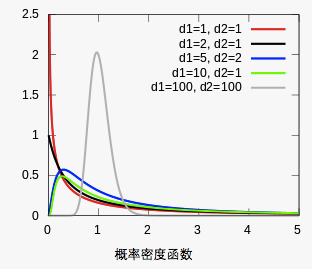$F = \frac { S _ { 大 } ^ { 2 } } { S _ { 小 } ^ { 2 } }$

$\begin{array} { l } { S _ { n _ { 1 } - 1 } ^ { 2 } = \frac { n _ { 1 } } { n _ { 1 } - 1 } S _ { 1 } ^ { 2 } = \frac { 12 } { 11 } \times 1.75 ^ { 2 } = 3.34 } \\ { S _ { n _ { 2 } - 1 } ^ { 2 } = \frac { n _ { 2 } } { n _ { 2 } - 1 } S _ { 2 } ^ { 2 } = \frac { 8 } { 7 } \times 1.73 ^ { 2 } = 3.42 } \\ { F = \frac { S _ { n _ { 2 } - 1 } ^ { 2 } } { S _ { n _ { 1 } - 1 } ^ { 2 } } = \frac { 3.42 } { 3.34 } = 1.02 } \end{array}$